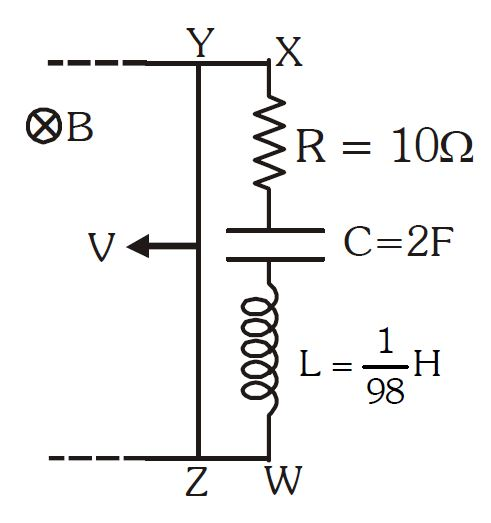# Maximum current in circuit!In the figure shown magnetic field $B$ is constant and uniform and in the direction perpendicular to the plane of paper. Velocity of a conducting wire $YZ$ is varying with time as $v = v_0 \ \cos (\omega t)$. Find the value of $\omega$ (in $\text{rad/sec}$) so that heat generated in circuit will be maximum (neglect friction and resistance of rails).

×